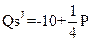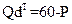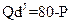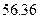Chapter 34, Problem 55P### Principles of Economics 2e

2nd Edition
Steven A. Greenlaw; David Shapiro
ISBN: 9781947172364

#### Solutions

Chapter
Section### Principles of Economics 2e

2nd Edition
Steven A. Greenlaw; David Shapiro
ISBN: 9781947172364
Textbook Problem

# Assume two countries, Thailand (T) and Japan (J), have one good: cameras. The demand (d) and supply (s) for cameras In Thailand and Japan is described by the following functions:P is the price measured in a common currency used in both countries, such as the Thai Baht.a. Compute the equilibrium price (P) and quantities in each country without trade.b. Now assume that free trade occurs. The free-trade price goes toBaht. Who exports and Imports cameras and in what quantities?

a)

To determine

The equilibrium quantities and prices of camera in countries Japan and Thailand, given the demand and supply of camera in each country.

Explanation

We are given the demand and supply of cameras in Japan and Thailand as follows:

QdT = 60 − P

QsT = −5 + 1/4P

QdJ = 80 − P

QsJ = −10 + 1/2P

i)Equilibrium in the market for cameras in Japan :

Equilibrium is defined as the situation where market forces of demand and supply are balanced. That is, equilibrium is achieved where quantity demanded is equal to quantity supplied.

Equating demand and supply in the market for cameras in Japan, we get,

sw QdJ=80 - PQsJ= -10+12PQdJ= QsJ80 - P = -10 +12P32P=90PJ&*#x00A0;= 60SubstitutingPJ*inQdJ,wegettheequilibriumoutputQdJ=80 - PQdJ=80 - 60QJ&*#x00A0;= 20

Graphical representation of the equilibrium in the market for cameras in Japan is as follows:

b)

To determine

The post trade import and export quantities, given the free trade price goes to 56.36 Baht.

### Still sussing out bartleby?

Check out a sample textbook solution.

See a sample solution

#### The Solution to Your Study Problems

Bartleby provides explanations to thousands of textbook problems written by our experts, many with advanced degrees!

Get Started